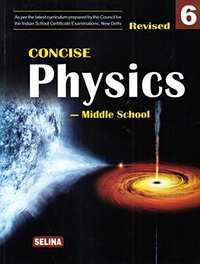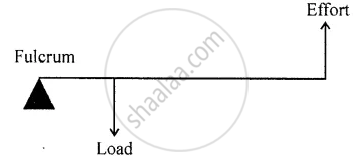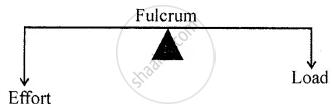ICSE Class 6CISCE
Share

Selina solutions for Class 6 Physics chapter 4 - Simple Machines

Selina Concise Physics - Middle School for Class 6 (2018-19 Session)

Selina Selina Concise Physics - Middle School Class 6 (2018-19 Session)Chapter 4: Simple Machines

Chapter 4: Simple Machines solutions [Page 0]

State whether the following statements is True or False.

A boy does work while pushing a wall.

State whether the following statements is True or False.

A machine performs work by itself.

State whether the following statements is True or False.

In an ideal machine, work done on load is equal to the work done by effort.

State whether the following statements is True or False.

All levers are force multipliers.

State whether the following statements is True or False.

A pulley changes the direction of force.

State whether the following statements is True or False.

An inclined plane always has the mechanical advantage more than 1.

Fill in the blank :

The useful work done by an actual machine is always ............ than the work done on the machine.

Fill in the blank :

In class II levers, the load is in between fulcrum and .........

Fill in the blank :

The mechanical advantage of class .......lever is always less than 1.

Fill in the blank :

A pulley is used to change .....................................

Fill in the blank :

Mechanical advantage of an inclined plane is always ..........................

Match the following :

 Column A Column B (a) Needle (1) class II lever (b) Door knob (2) inclined plane (c) Ramp (3) Class I lever (d) Lemon crusher (4) Wheel and axle (e) See saw (5) wedge

Select the correct alternative :

For an ideal machine, the efficiency is

1. greater than unity
2. less thaii unity
3. equalto unity
4. depends on the value of load

Select the correct alternative :

Mechanical advantage of a machine is defined as:

1. Load X Effort
2. Load / Effort
3. Load + Effort
4. Effort / Load

Select the correct alternative :

The mechanical advantage of a lever is equal to:

1. Load arm / Effort arm
2. Effort arm / Load arm
3. Load arm + Effortarm
4. Load ann — Effort arm

Select the correct alternative :

A pulley is used because it

1. has the mechanical advantage greatcr than one
2. has 100% efficiency
3. helps to apply the force in a convenient direction
4. requires more effort to raise a less load.

Select the correct alternative :

Wheel is used with axle because

1. sliding friction is less than the roffing friction
2. rolling friction is less than the sliding friction
3. they work as the inclined plane
4. They help us to change the direction of force.

Chapter 4: Simple Machines solutions [Page 0]

When is work said to be done by a force ?

What is energy ?

What do you understand by a machine ?

What is the principle on which a machine works ?

State two functions of a machine.

Name six simple machines. Give an example of each machine.

Define the term ‘work input’ and ‘work output’ in relation to a machine.

Explain the term mechanical advantage of a machine.

Define the term efficiency of a machine.

What is an ideal machine ?

Can a machine have an efficiency of 100% ? Give a reason to support your answer.

A machine is 75% efficient’. What do you understand by this statement ?

What is a lever ?

Describe three orders of levers giving an example of each. Draw neat diagrams showing the positions of fulcrum, load and effort in each kind of lever.

What do you mean by the mechanical advantage of a lever ?

Which class of lever has the mechanical advantage always more than 1 ? Give an example.

Which class of lever has the mechanical advantage always less than 1 ? Give an example.

Give one example of class I lever in case where the mechanical advantage is more than 1 ?

Give one example of class I lever in case where the mechanical advantage is equal to 1 ?

Give one example of class I lever in case where the mechanical advantage is less than 1 ?

Name the class to which the following lever belong:

A pair of scissors ?

Name the class to which the following lever belong:

A lemom squeezer ?

Name the class to which the following lever belong:

A nut cracker  ?

Name the class to which the following lever belong:

A pair of suger tongs ?

Name the class to which the following lever belong:

A beam balance ?

Name the class to which the following lever belong:

An oar rowing a boat ?

Name the class to which the following lever belong:

A wheel barrow ?

Name the class to which the following lever belong:

A see saw ?

Name the class to which the following levers belong:

A pair of pilers  ?

Name the class to which the following lever belong:

A crow bar ?

The diagram given below shows the three kinds of levers. Name the class of each lever and give one example of each class .Draw diagrams to illustrate the positions of fulcrum, load and effort, in the following:

A see saw .

Draw diagrams to illustrate the position of fulcrum, load and effort, in the following a beam balance .

Draw diagrams to illustrate the position of fulcrum, load and effort, in the following a nut cracker ?

Draw diagrams to illustrate the position of fulcrum, load and effort, in the following a pair of forceps ?

How can you increase the mechanical advantage of a lever ?

How does the friction at the fulcrum affect the mechanical advantage of the lever ?

State three differences between the three classes of levers .

What is a pulley ?

What is the mechanical advantage of an ideal pulley ?

The mechanical advantage of an actual pulley is less than 1. Give a reason.

What is the justification for using the pulley then ?

Draw a neat labelled diagram showing a pulley being used to lift a load. How are load and effort related in an ideal situation?

What is an inclined plane?

What is the use of  inclined plane ? Give two examples where it is used.

What is a screw ? Give two examples.

What is wheel and axle ? Give two examples.

How does a wheel help in moving the axle ?

What is a wedge ? Give two examples.

Name the machine to which the following belong :

Beam balance

Name the machine to which the following belong :

Lemon crusher

Name the machine to which the following belong :

Sugar tongs

Name the machine to which the following belong :

Ramp

Name the machine to which the following belong:

Door knob

Name the machine to which the following belong :

Needle

What care would you take to increase the life span of a machine which you use ?

Select the correct statement :
(a) A wheel barrow is a lever of class I.
(b) The efficiency of a machine is always 100%
(c) Friction in moving parts of a machine reduces its efficiency.
(d) No lever has the mechanical advantage greater than 1.
(e) It is easier to lift a load vertically up than to push it along an inclined plane.
(f) A screw is made by two inclined planes placed together.

Chapter 4: Simple Machines solutions [Page 0]

In a machine an effort of 10 kgf is applied to lift a load of 100 kgf. What is its mechanical advantage ?

The mechanical advantage of a machine is 5. How much load it can exert for the effort of 2 kgf ?

The mechanical advantage of a machine is 2. It is used to raise a load of 15 kgf. What effort is needed ?

A lever of length 100 cm has effort of 15 kgf at a distance of 40 cm from the fulcrum at one end. What load can be applied at its other end ?

In a lever, fulcrum is at one end at a distance of 30 cm from the load and effort is at the other end at a distance of 90 cm from the load. Find :
(a) the length of load arm,
(b) the length of effort arm, and
(c) the mechanical advantage of the lever.

Chapter 4: Simple Machines solutions [Page 0]

What is a machine ?

What do you understand by a complex machine ?

Name the simplest of all types of machines.

State the principle of levers.

What is a Class I lever ?

Chapter 4: Simple Machines solutions [Page 0]

Tick the most appropriate answer.

The force applied on a machine to do work is called the

2. effort
3. efficiency
4. fulcrum

Tick the most appropriate answer.

If the effort lies between the fulcrum and the load, then the lever belongs to which class ?

1. Class I
2. Class II
3. Class III
4. Class IV

Tick the most appropriate answer.

Which of the following is a Class II lever ?

1. Pliers
2. A beam balance
3. A nut-cracker
4. A knife

Tick the most appropriate answer.

A pair of scissors is an example of a/an

1. wedge
2. lever
3. inclined plane
4. screw

Tick the most appropriate answer.

The mechanical advantage of an inclined plane is always

1. greater than 1
2. less than 1
3. equal to 1
4. zero

Tick the most appropriate answer.

The effort required to lift a load of 800 N by using a lever having a mechanical advantage of 1.6 is

1. 1080 N
2. 240 N
3. 720 N
4. 500 N

Tick the most appropriate answer

A machine made up of two or more sloping surface is known as a

1. wedge
2. screw
3. pulley
4. lever

Chapter 4: Simple Machines solutions [Page 0]

State if the following statement is true or false.

There are four types of simple machines.

State if the following statement is true or false.

The load and effort can act at a single point in a lever.

State if the following statement is true or false.

A screw is a special case of an inclined plane.

State if the following statement is true or false :

The effort required to insert a screw into wood is less than that needed to insert a nail into wood .

State if the following statement is true or false :

A single movable pulley is a pulley that has its axis of rotation fixed.

State if the following statement is true or false :

A rotation spindle tap is an example of a wheel-and-axle arrangement.

State if the following statement is true or false.

A sewing needle is a wedge type simple machine.

State if the following statement is true or false.

Work done by a machine is always more than the work done on a machine.

Chapter 4: Simple Machines solutions [Page 0]

Given an example of a Class I lever.

Which type of machine is used to squeeze a lemon ?

Write the relationship between mechanical advantage, load and effort.

Name the type of machine made by putting two inclined planes together.

Give one example of a machine used to multiply speed.

Write the formula for calculating the efficiency of a machine.

Chapter 4: Simple Machines solutions [Page 0]

Explain the various functions that a machine can perform.

What is the basis of classification of levers ?

What is the function of a screw ? Give any one use of a screw.

Using a suitable example, describe how a machine acts as a force multiplier.

What do you understand by the term efficiency of a machine’?

Mention any two methods by which we can take care of machines.

Chapter 4: Simple Machines solutions [Page 0]

Draw simplified diagrams by clearly showing the position of load, effort and fulcrum for Class I, Class II and Class III levers.

Differentiate between a single fixed pulley and a single movable pulley.

How does a pulley make work simpler ?

What is a wedge ? Explain the principle on which it works by giving suitable examples.

What is an inclined plane ?

What is the use of an inclined plane ?

Chapter 4: Simple Machines solutions [Page 0]

Machines are able to make our work convenient.

The efficiency of a machine is always less than 100%.

The front end of a boat is shaped like a wedge.

Chapter 4: Simple Machines solutions [Page 0]

The length of a lever is 2 m. Calculate its mechanical advantage if the fulcrum is situated at a distance of 40 cm from the effort.

The length of the load arm of a lever is 6 m long and the effort arm is 3 m long. What is the effort required to lift a load of 40 N ?

Calculate the mechanical advantage of a crowbar of length 240 cm if its fulcrum is situated at a distance of 40 cm from the load.

What effort will be required to lift a load of 500 N by a single movable pulley ? [Hint: Mechanical advantage of a single movable pulley is two].

Chapter 4: Simple Machines

Selina Selina Concise Physics - Middle School Class 6 (2018-19 Session)Selina solutions for Class 6 Physics chapter 4 - Simple Machines

Selina solutions for Class 6 Physics chapter 4 (Simple Machines) include all questions with solution and detail explanation. This will clear students doubts about any question and improve application skills while preparing for board exams. The detailed, step-by-step solutions will help you understand the concepts better and clear your confusions, if any. Shaalaa.com has the CISCE Selina Concise Physics - Middle School for Class 6 (2018-19 Session) solutions in a manner that help students grasp basic concepts better and faster.

Further, we at Shaalaa.com are providing such solutions so that students can prepare for written exams. Selina textbook solutions can be a core help for self-study and acts as a perfect self-help guidance for students.

Concepts covered in Class 6 Physics chapter 4 Simple Machines are Concept of Energy, Simple Machines - Basic Concept, Simple Machines - Mechanical Advantage, Types of Simple Machines - Lever, Types of Simple Machines - Wheel and Axle, Types of Simple Machines - Pulley, Types of Simple Machines - Inclined Plane, Types of Simple Machines - Wedge, Types of Simple Machines - Screw, Different Orders of Levers, Numerical Based on Mechanical Advantage Or Leverage - Load × Load Arm = Effort × Effort Arm..

Using Selina Class 6 solutions Simple Machines exercise by students are an easy way to prepare for the exams, as they involve solutions arranged chapter-wise also page wise. The questions involved in Selina Solutions are important questions that can be asked in the final exam. Maximum students of CISCE Class 6 prefer Selina Textbook Solutions to score more in exam.

Get the free view of chapter 4 Simple Machines Class 6 extra questions for Physics and can use Shaalaa.com to keep it handy for your exam preparation

S